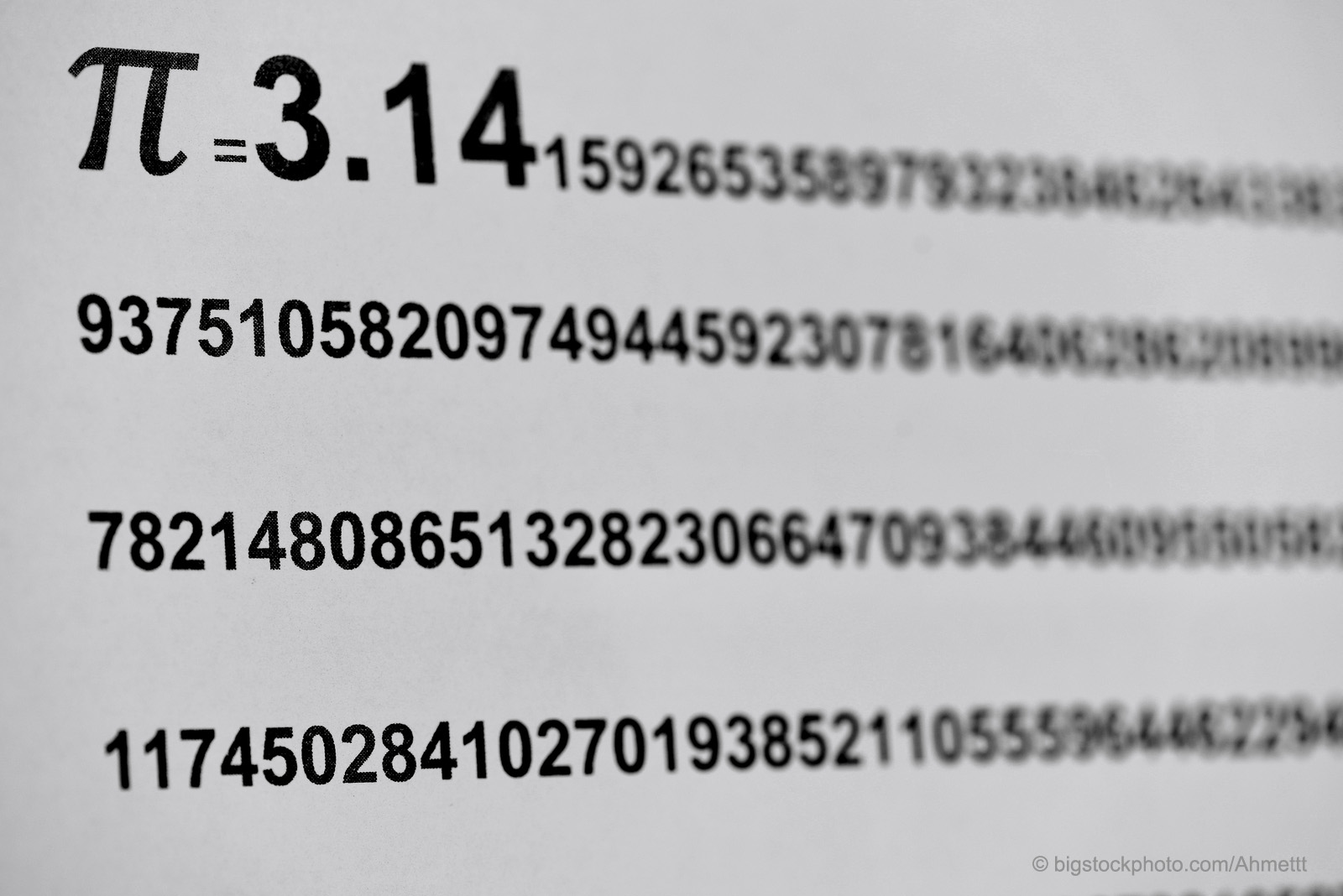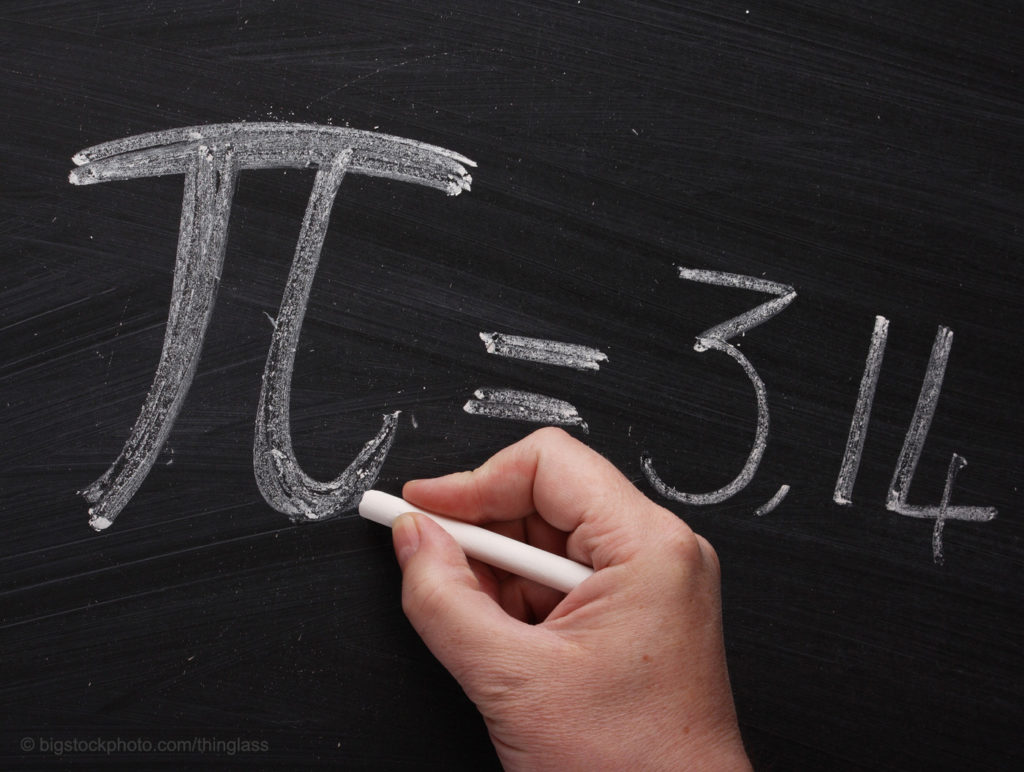## Value of Pi in the BibleIt may not appear that the passage below would cause a major question of biblical infallibility. However, atheists and skeptics try to make an issue of the value of pi in the Bible.

Also he made a molten sea of ten cubits from brim to brim, round in compass, and five cubits the height thereof; and a line of thirty cubits did compass it round about. 2 Chronicles 4:2

In several lectureships recently, I have been challenged to explain why the passage is not in error. The point is that it gives the diameter of the top of the circular bowl and its circumference. If the bowl’s diameter is known, one can calculate the circumference by the formula we all learned in elementary school, which says that C=2πr (the circumference is equal to two times pi times the radius). If we know the circumference, we can calculate the value of π, which would be C divided by 2r. In this case that gives the value of π as 3.00000. We all know that π is twenty-two sevenths or 3.142857142. Obviously, according to the skeptics, the value of pi in the Bible is in error.

My first reaction to hearing this challenge was that it was nit-picking in the first degree. I have seen it repeated so many times by so many recently that I need to answer it again. It may also be that this passage is actually a positive proof of the integrity and accuracy of the biblical narrative.

The first point that I need to make is that one must observe significant figures when making any measurement. In my physics laboratory, the balances measure things to a tenth of a gram. If you wish to measure the mass of something to a hundredth or thousandth of a gram, you have to use another balance. Every year, I have a student who wants to record a mass accurate to a millionth of a gram. On their lab sheet, they will give me a number like 28.6581432 grams—on a balance that has no marks or calibrations beyond a tenth of a gram!

In the case of the biblical record, the unit is the cubit. A cubit is the distance from the elbow to the tip of the finger. It should be evident that the length of the cubit depends upon the size of the person whose forearm you measure. My wife’s cubit is about three inches shorter than mine. This is no factor in the kind of measurements that the cubit was used for, but it does mean that the cubit was only accurate to the nearest whole number—three. Obviously, the value of pi in the Bible is accurate to the number of significant figures to which the cubit can be measured.

It is also possible that the 30-cubit measurement was around the inside circumference of the bowl since that is what held the liquid. The 10 cubits may be the outside diameter since that would be what the bowl was forged from and the space it occupied. In that case, the outside circumference would be 31.43. If we assume the same man made both measurements and was accurate to two decimal places (which is unlikely), we can calculate the thickness of the material of which the bowl was made. Since C=2πr, then:

r(outside) – r(inside) = C(out) – C(in) =.18 cubits

That is about 3 inches which would mean the thickness of the bowl would be 1.5 inches, which is very reasonable. I made this point at a lecture recently and had an atheist respond with, “I don’t care what’s reasonable, the fact is, the Bible says pi is 3.00 and that’s wrong!” The idea she was advancing was that if there was any possible way she could interpret a biblical passage that would make it look ridiculous, that is the way she would interpret it. No amount of discussion about significant figures or possible measurement parameters would change her mind. She insisted the value of pi in the Bible is 3.000 and that is wrong!

The same person became incensed when I would not accept the dubious possibility that volcanoes produced the layers of iridium found in the dinosaur remains. This idea, which deals with evolution, has a mountain of other evidence which indicates that volcanoes did not produce these layers, but that they came from an astronomical catastrophe.

I have had the same kind of experience with religious people when their pet religious tradition was called into question by the available evidence. Certainly, this discussion points out some questions about approaches. Perhaps we have to get to the realization that putting pearls before swine is an exercise in futility. (See Matthew 7:6.)There is no limit to the extremes that skeptics will go trying to find mistakes in the Bible. One recent case involves cubit pi. An atheist claimed that the Bible was full of mathematical mistakes and impossibilities indicating the primitive nature and lack of knowledge of the authors. The case used was 1 Kings 7:23-26 and 2 Chronicles 4:2,5 where a laver is described, and numbers are given for its dimensions in cubits. The Bible says that the circumference of the round laver was 30 cubits and that the diameter was 10 cubits from rim to rim. The formula for the circumference of a circle is pi (3.14) times the diameter, so in this case it would be 31.4 cubits, not 30. A Hebrew cubit was 17.5 inches, a Babylonian cubit was 19.8 inches, and an Egyptian cubit was 20.63 inches, so this seems to be about a 19-inch mistake.